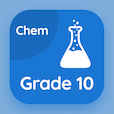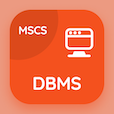Computer Science Online Courses

C++ Quizzes

C++ Quiz PDF - Complete

# Initializing in Declaration Quiz Questions Online p. 115

Learn Initializing in Declaration quiz questions and answers, initializing in declaration MCQ with answers PDF 115 to learn C++ online course. Introduction to C++ Programming trivia questions, Initializing in Declaration Multiple Choice Questions (MCQ) for online college degrees. "Initializing in Declaration Quiz" PDF Book: pointers and references, data hierarchy, character and string literals, increment decrement operator, initializing in declaration test prep for online bachelor's degree computer science.

"Which statement is correct for chained assignment?" MCQ PDF: y=x=22;, y=22;, y=(x=22);, and both b and c for cheapest online computer science degree. Study introduction to c++ programming questions and answers to improve problem solving skills for computer majors.

## Quiz on Initializing in Declaration MCQs

MCQ: Which statement is correct for chained assignment?

y=22;
y=x=22;
y=(x=22);
Both B and C

MCQ: Input stream Cin reads the items form

Right to left
Left to right
Middle to end
None of them

MCQ: The length of a string literal is determined by

Changing it into the integer type
Putting it into an array
The number of characters it contain
None of them

MCQ: A field consisting of decimal digits could represents a

person's name
person's age
person's father name
person's age

MCQ: The address operator is denoted by

@
\$
#
&

### More Quizzes from C++ Course